# 10.3 Polar coordinates  (Page 5/8)

 Page 5 / 8

How are the polar axes different from the x - and y -axes of the Cartesian plane?

Explain how polar coordinates are graphed.

Determine $\text{\hspace{0.17em}}\theta \text{\hspace{0.17em}}$ for the point, then move $\text{\hspace{0.17em}}r\text{\hspace{0.17em}}$ units from the pole to plot the point. If $\text{\hspace{0.17em}}r\text{\hspace{0.17em}}$ is negative, move $\text{\hspace{0.17em}}r\text{\hspace{0.17em}}$ units from the pole in the opposite direction but along the same angle. The point is a distance of $\text{\hspace{0.17em}}r\text{\hspace{0.17em}}$ away from the origin at an angle of $\text{\hspace{0.17em}}\theta \text{\hspace{0.17em}}$ from the polar axis.

How are the points $\text{\hspace{0.17em}}\left(3,\frac{\pi }{2}\right)\text{\hspace{0.17em}}$ and $\text{\hspace{0.17em}}\left(-3,\frac{\pi }{2}\right)\text{\hspace{0.17em}}$ related?

Explain why the points $\text{\hspace{0.17em}}\left(-3,\frac{\pi }{2}\right)\text{\hspace{0.17em}}$ and $\text{\hspace{0.17em}}\left(3,-\frac{\pi }{2}\right)\text{\hspace{0.17em}}$ are the same.

The point $\text{\hspace{0.17em}}\left(-3,\frac{\pi }{2}\right)\text{\hspace{0.17em}}$ has a positive angle but a negative radius and is plotted by moving to an angle of $\text{\hspace{0.17em}}\frac{\pi }{2}\text{\hspace{0.17em}}$ and then moving 3 units in the negative direction. This places the point 3 units down the negative y -axis. The point $\text{\hspace{0.17em}}\left(3,-\frac{\pi }{2}\right)\text{\hspace{0.17em}}$ has a negative angle and a positive radius and is plotted by first moving to an angle of $\text{\hspace{0.17em}}-\frac{\pi }{2}\text{\hspace{0.17em}}$ and then moving 3 units down, which is the positive direction for a negative angle. The point is also 3 units down the negative y -axis.

## Algebraic

For the following exercises, convert the given polar coordinates to Cartesian coordinates with $\text{\hspace{0.17em}}r>0\text{\hspace{0.17em}}$ and $\text{\hspace{0.17em}}0\le \theta \le 2\pi .\text{\hspace{0.17em}}$ Remember to consider the quadrant in which the given point is located when determining $\text{\hspace{0.17em}}\theta \text{\hspace{0.17em}}$ for the point.

$\left(7,\frac{7\pi }{6}\right)$

$\left(5,\pi \right)$

$\left(-5,0\right)$

$\left(6,-\frac{\pi }{4}\right)$

$\left(-3,\frac{\pi }{6}\right)$

$\left(-\frac{3\sqrt{3}}{2},-\frac{3}{2}\right)$

$\left(4,\frac{7\pi }{4}\right)$

For the following exercises, convert the given Cartesian coordinates to polar coordinates with $\text{\hspace{0.17em}}r>0,\text{\hspace{0.17em}}\text{\hspace{0.17em}}0\le \theta <2\pi .\text{\hspace{0.17em}}$ Remember to consider the quadrant in which the given point is located.

$\left(4,2\right)$

$\left(-4,6\right)$

$\left(3,-5\right)$

$\left(\sqrt{34},5.253\right)$

$\left(-10,-13\right)$

$\left(8,8\right)$

$\left(8\sqrt{2},\frac{\pi }{4}\right)$

For the following exercises, convert the given Cartesian equation to a polar equation.

$x=3$

$y=4$

$r=4\mathrm{csc}\theta$

$y=4{x}^{2}$

$y=2{x}^{4}$

$r=\sqrt{\frac{sin\theta }{2co{s}^{4}\theta }}$

${x}^{2}+{y}^{2}=4y$

${x}^{2}+{y}^{2}=3x$

$r=3\mathrm{cos}\theta$

${x}^{2}-{y}^{2}=x$

${x}^{2}-{y}^{2}=3y$

$r=\frac{3\mathrm{sin}\theta }{\mathrm{cos}\left(2\theta \right)}$

${x}^{2}+{y}^{2}=9$

${x}^{2}=9y$

$r=\frac{9\mathrm{sin}\theta }{{\mathrm{cos}}^{2}\theta }$

${y}^{2}=9x$

$9xy=1$

$r=\sqrt{\frac{1}{9\mathrm{cos}\theta \mathrm{sin}\theta }}$

For the following exercises, convert the given polar equation to a Cartesian equation. Write in the standard form of a conic if possible, and identify the conic section represented.

$r=3\mathrm{sin}\text{\hspace{0.17em}}\theta$

$r=4\mathrm{cos}\text{\hspace{0.17em}}\theta$

${x}^{2}+{y}^{2}=4x\text{\hspace{0.17em}}$ or $\text{\hspace{0.17em}}\frac{{\left(x-2\right)}^{2}}{4}+\frac{{y}^{2}}{4}=1;$ circle

$r=\frac{4}{\mathrm{sin}\text{\hspace{0.17em}}\theta +7\mathrm{cos}\text{\hspace{0.17em}}\theta }$

$r=\frac{6}{\mathrm{cos}\text{\hspace{0.17em}}\theta +3\mathrm{sin}\text{\hspace{0.17em}}\theta }$

$3y+x=6;\text{\hspace{0.17em}}$ line

$r=2\mathrm{sec}\text{\hspace{0.17em}}\theta$

$r=3\mathrm{csc}\text{\hspace{0.17em}}\theta$

$y=3;\text{\hspace{0.17em}}$ line

$r=\sqrt{r\mathrm{cos}\text{\hspace{0.17em}}\theta +2}$

${r}^{2}=4\mathrm{sec}\text{\hspace{0.17em}}\theta \text{\hspace{0.17em}}\mathrm{csc}\text{\hspace{0.17em}}\theta$

$xy=4;\text{\hspace{0.17em}}$ hyperbola

$r=4$

${r}^{2}=4$

${x}^{2}+{y}^{2}=4;\text{\hspace{0.17em}}$ circle

$r=\frac{1}{4\mathrm{cos}\text{\hspace{0.17em}}\theta -3\mathrm{sin}\text{\hspace{0.17em}}\theta }$

$r=\frac{3}{\mathrm{cos}\text{\hspace{0.17em}}\theta -5\mathrm{sin}\text{\hspace{0.17em}}\theta }$

$x-5y=3;\text{\hspace{0.17em}}$ line

## Graphical

For the following exercises, find the polar coordinates of the point.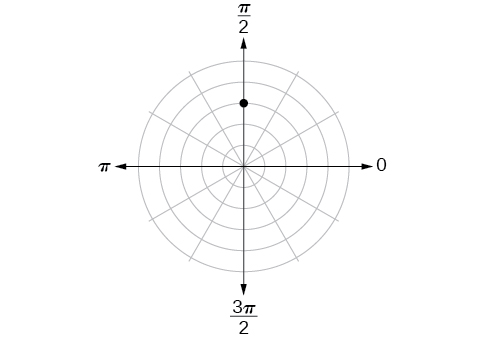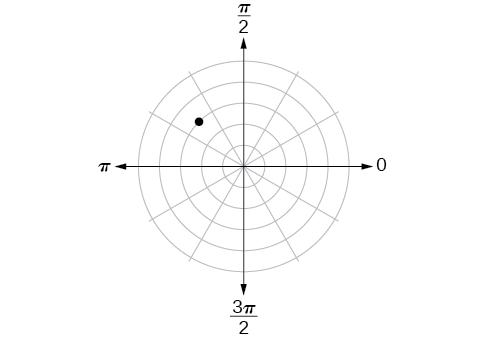$\left(3,\frac{3\pi }{4}\right)$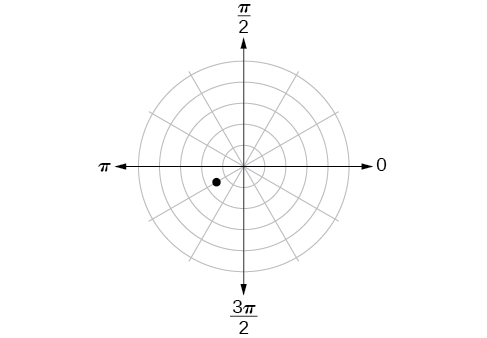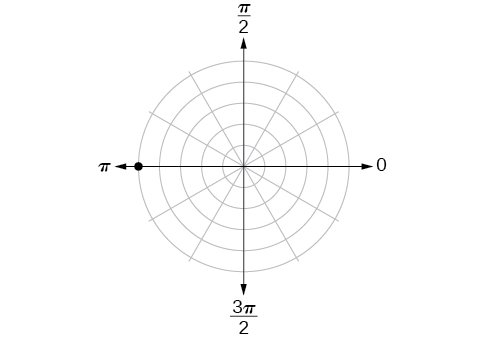$\left(5,\pi \right)$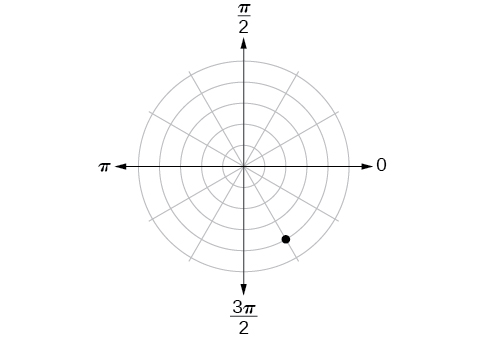For the following exercises, plot the points.

$\left(-2,\frac{\pi }{3}\right)$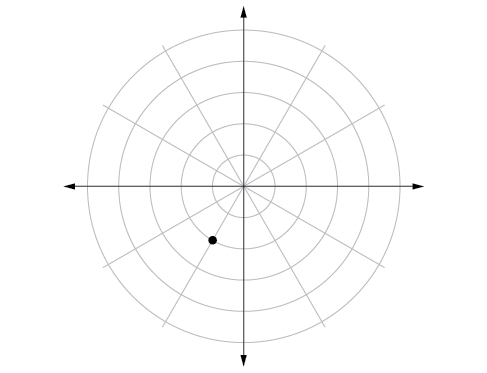$\left(-1,-\frac{\pi }{2}\right)$

$\left(3.5,\frac{7\pi }{4}\right)$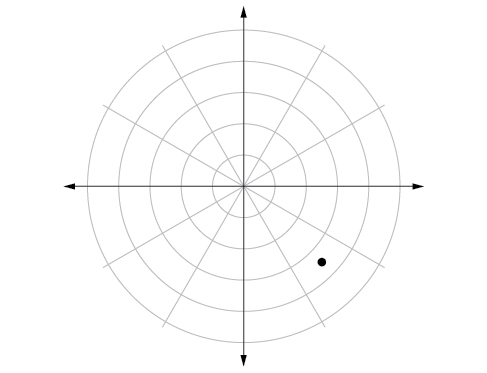$\left(-4,\frac{\pi }{3}\right)$

$\left(5,\frac{\pi }{2}\right)$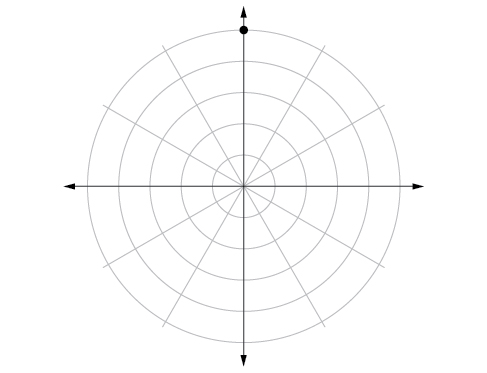$\left(4,\frac{-5\pi }{4}\right)$

$\left(3,\frac{5\pi }{6}\right)$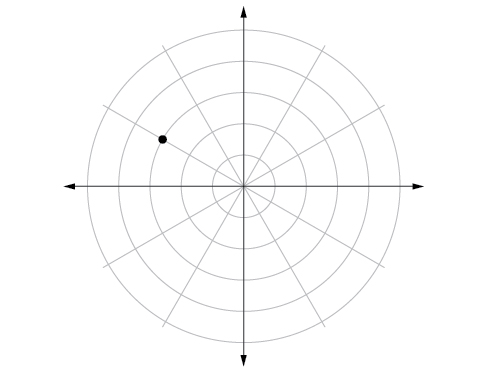$\left(-1.5,\frac{7\pi }{6}\right)$

$\left(-2,\frac{\pi }{4}\right)$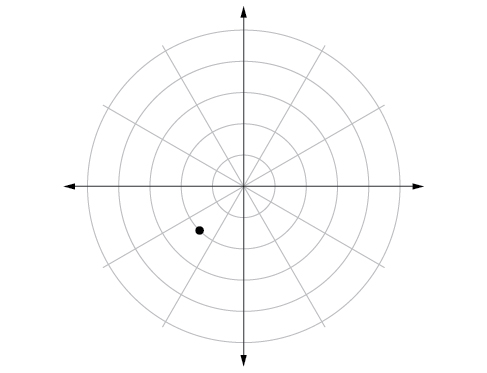$\left(1,\frac{3\pi }{2}\right)$

For the following exercises, convert the equation from rectangular to polar form and graph on the polar axis.

$5x-y=6$

$r=\frac{6}{5\mathrm{cos}\theta -\mathrm{sin}\theta }$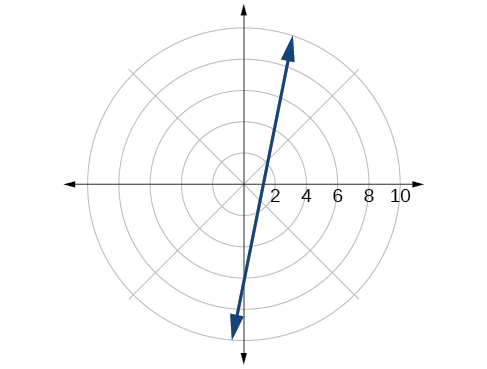$2x+7y=-3$

${x}^{2}+{\left(y-1\right)}^{2}=1$

$r=2\mathrm{sin}\theta$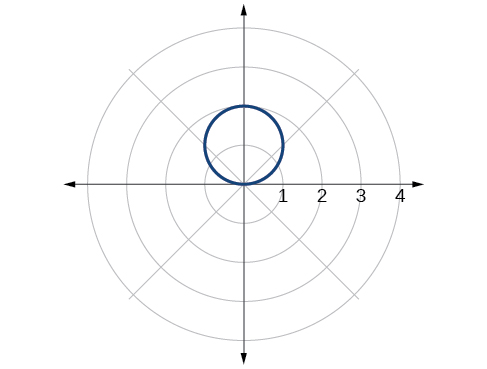${\left(x+2\right)}^{2}+{\left(y+3\right)}^{2}=13$

$x=2$

$r=\frac{2}{\mathrm{cos}\theta }$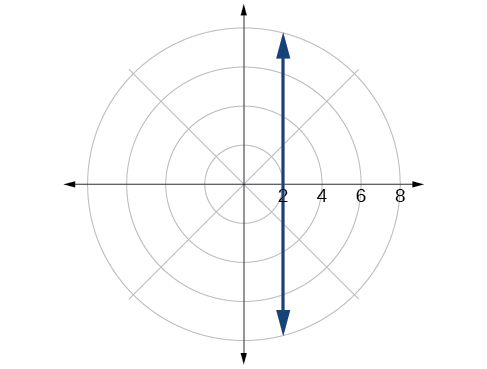${x}^{2}+{y}^{2}=5y$

${x}^{2}+{y}^{2}=3x$

$r=3\mathrm{cos}\theta$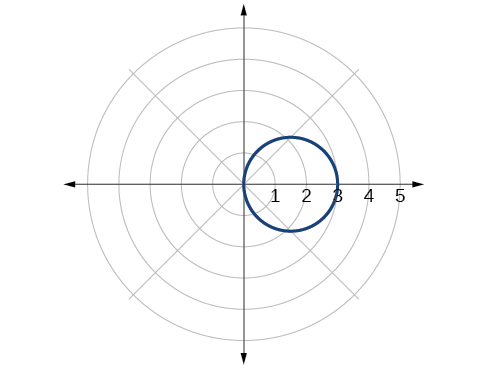For the following exercises, convert the equation from polar to rectangular form and graph on the rectangular plane.

$r=6$

$r=-4$

${x}^{2}+{y}^{2}=16$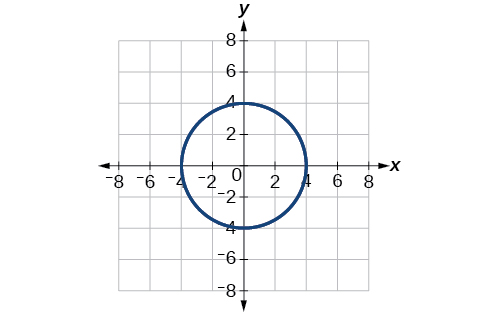$\theta =-\frac{2\pi }{3}$

$\theta =\frac{\pi }{4}$

$y=x$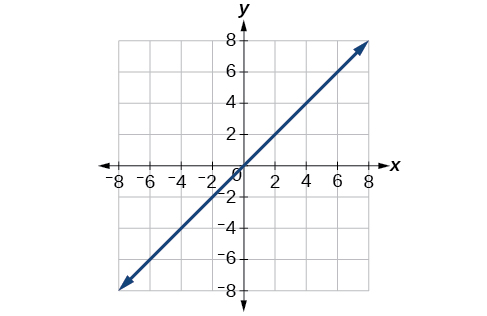$r=\mathrm{sec}\text{\hspace{0.17em}}\theta$

$r=-10\mathrm{sin}\text{\hspace{0.17em}}\theta$

${x}^{2}+{\left(y+5\right)}^{2}=25$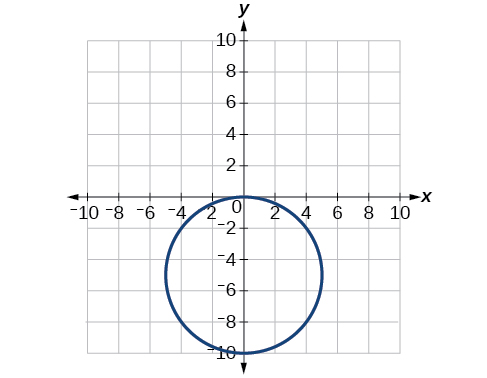$r=3\mathrm{cos}\text{\hspace{0.17em}}\theta$

## Technology

Use a graphing calculator to find the rectangular coordinates of $\text{\hspace{0.17em}}\left(2,-\frac{\pi }{5}\right).\text{\hspace{0.17em}}$ Round to the nearest thousandth.

$\left(1.618,-1.176\right)$

Use a graphing calculator to find the rectangular coordinates of $\text{\hspace{0.17em}}\left(-3,\frac{3\pi }{7}\right).\text{\hspace{0.17em}}$ Round to the nearest thousandth.

Use a graphing calculator to find the polar coordinates of $\text{\hspace{0.17em}}\left(-7,8\right)\text{\hspace{0.17em}}$ in degrees. Round to the nearest thousandth.

$\left(10.630,131.186°\right)$

Use a graphing calculator to find the polar coordinates of $\text{\hspace{0.17em}}\left(3,-4\right)\text{\hspace{0.17em}}$ in degrees. Round to the nearest hundredth.

Use a graphing calculator to find the polar coordinates of $\text{\hspace{0.17em}}\left(-2,0\right)\text{\hspace{0.17em}}$ in radians. Round to the nearest hundredth.

$\text{\hspace{0.17em}}\left(2,3.14\right)or\left(2,\pi \right)\text{\hspace{0.17em}}$

## Extensions

Describe the graph of $\text{\hspace{0.17em}}r=a\mathrm{sec}\text{\hspace{0.17em}}\theta ;a>0.$

Describe the graph of $\text{\hspace{0.17em}}r=a\mathrm{sec}\text{\hspace{0.17em}}\theta ;a<0.$

A vertical line with $\text{\hspace{0.17em}}a\text{\hspace{0.17em}}$ units left of the y -axis.

Describe the graph of $\text{\hspace{0.17em}}r=a\mathrm{csc}\text{\hspace{0.17em}}\theta ;a>0.$

Describe the graph of $\text{\hspace{0.17em}}r=a\mathrm{csc}\text{\hspace{0.17em}}\theta ;a<0.$

A horizontal line with $\text{\hspace{0.17em}}a\text{\hspace{0.17em}}$ units below the x -axis.

What polar equations will give an oblique line?

For the following exercise, graph the polar inequality.

$r<4$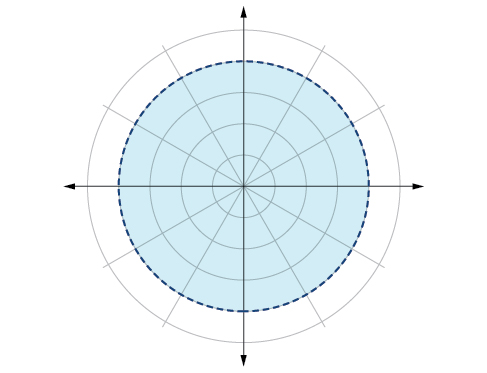$0\le \theta \le \frac{\pi }{4}$

$\theta =\frac{\pi }{4},\text{\hspace{0.17em}}r\text{\hspace{0.17em}}\ge \text{\hspace{0.17em}}2$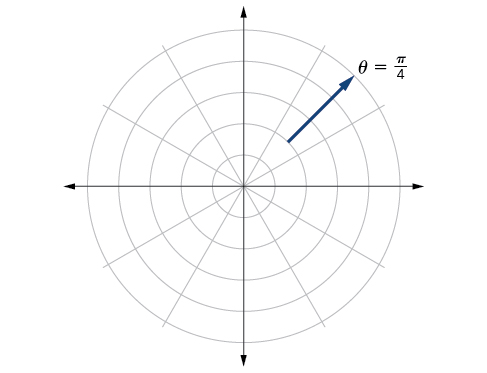$\theta =\frac{\pi }{4},\text{\hspace{0.17em}}r\text{\hspace{0.17em}}\ge -3$

$0\le \theta \le \frac{\pi }{3},\text{\hspace{0.17em}}r\text{\hspace{0.17em}}<\text{\hspace{0.17em}}2$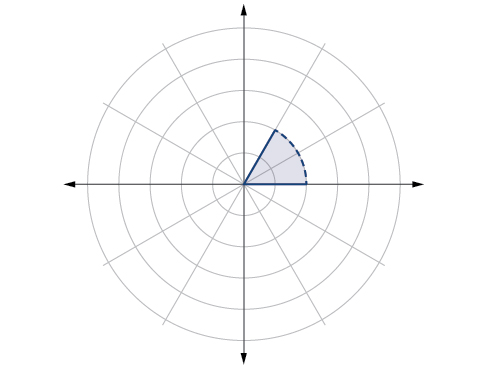$\frac{-\pi }{6}<\theta \le \frac{\pi }{3},-3

#### Questions & Answers

x exposant 4 + 4 x exposant 3 + 8 exposant 2 + 4 x + 1 = 0
x exposent4+4x exposent3+8x exposent2+4x+1=0
HERVE
How can I solve for a domain and a codomains in a given function?
ranges
EDWIN
Thank you I mean range sir.
Oliver
proof for set theory
don't you know?
Inkoom
find to nearest one decimal place of centimeter the length of an arc of circle of radius length 12.5cm and subtending of centeral angle 1.6rad
factoring polynomial
find general solution of the Tanx=-1/root3,secx=2/root3
find general solution of the following equation
Nani
the value of 2 sin square 60 Cos 60
0.75
Lynne
0.75
Inkoom
when can I use sin, cos tan in a giving question
depending on the question
Nicholas
I am a carpenter and I have to cut and assemble a conventional roof line for a new home. The dimensions are: width 30'6" length 40'6". I want a 6 and 12 pitch. The roof is a full hip construction. Give me the L,W and height of rafters for the hip, hip jacks also the length of common jacks.
John
I want to learn the calculations
where can I get indices
I need matrices
Nasasira
hi
Raihany
Hi
Solomon
need help
Raihany
maybe provide us videos
Nasasira
Raihany
Hello
Cromwell
a
Amie
What do you mean by a
Cromwell
nothing. I accidentally press it
Amie
you guys know any app with matrices?
Khay
Ok
Cromwell
Solve the x? x=18+(24-3)=72
x-39=72 x=111
Suraj
Solve the formula for the indicated variable P=b+4a+2c, for b
Need help with this question please
b=-4ac-2c+P
Denisse
b=p-4a-2c
Suddhen
b= p - 4a - 2c
Snr
p=2(2a+C)+b
Suraj
b=p-2(2a+c)
Tapiwa
P=4a+b+2C
COLEMAN
b=P-4a-2c
COLEMAN
like Deadra, show me the step by step order of operation to alive for b
John
A laser rangefinder is locked on a comet approaching Earth. The distance g(x), in kilometers, of the comet after x days, for x in the interval 0 to 30 days, is given by g(x)=250,000csc(π30x). Graph g(x) on the interval [0, 35]. Evaluate g(5)  and interpret the information. What is the minimum distance between the comet and Earth? When does this occur? To which constant in the equation does this correspond? Find and discuss the meaning of any vertical asymptotes.
The sequence is {1,-1,1-1.....} hasByByByBy Sam Luong